# Surface Area Of A Hemisphere

Here we will learn about the surface area of a hemisphere.

There are also surface area of a hemisphere worksheets based on Edexcel, AQA and OCR exam questions, along with further guidance on where to go next if you’re still stuck.

## What is the surface area of a hemisphere?

The surface area of a hemisphere is the area which covers the outer surface of a hemisphere.

To calculate this we need to know what a hemisphere is. A hemisphere is a three-dimensional object that is half of a sphere. The radius of the hemisphere is r.

To calculate the surface area of a sphere with radius r, we can use the formula

\text{Surface area of a sphere}=4\pi{r}^{2}.

We can then adapt this formula, to find the curved surface area of the hemisphere we need to halve the surface area of the sphere.

4\pi{r}^{2}\div 2=2\pi{r}^{2}

However this would only give the curved surface area.

In order to calculate the total surface area we need to add the curved surface area to the area of the base of the hemisphere. The base surface is a circle and to calculate the area of the circle we need to use the formula \pi r^{2}.

Therefore the total surface area of a hemisphere formula is

2\pi{r}^{2}+\pi{r}^{2}=3\pi{r}^{2}.

Here are the surface area of a hemisphere formulas,

\begin{aligned} &\text{Curved surface area}=2\pi{r}^{2} \\\\ &\text{Total surface area of a hemisphere}=3\pi{r}^{2} \end{aligned}

### What is the surface area of a hemisphere?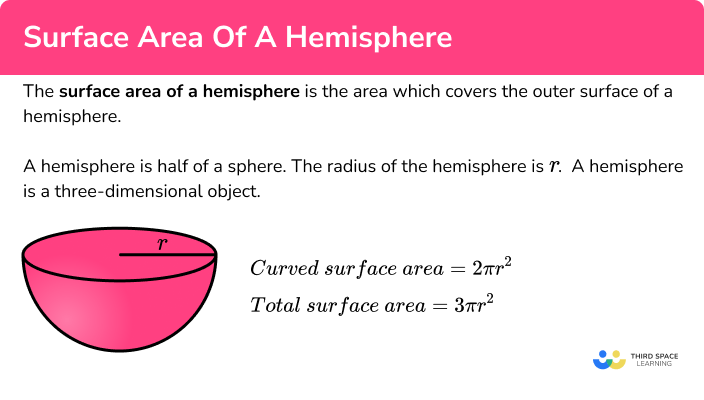## How to calculate the surface area of a hemisphere

In order to calculate the surface area of a hemisphere:

1. Write down the formula for the surface area of a sphere.
2. Find the curved surface area of the hemisphere.
3. If needed, find the total surface area.

### Explain how to calculate the surface area of a hemisphere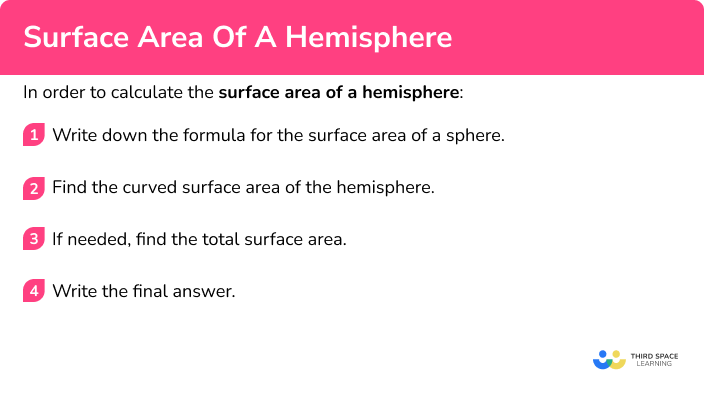## Related lessons on hemisphere shape

Surface area of a hemisphere is part of our series of lessons to support revision on hemisphere shape. You may find it helpful to start with the main hemisphere shape lesson for a summary of what to expect, or use the step by step guides below for further detail on individual topics. Other lessons in this series include:

## Surface area of a hemisphere examples

### Example 1: curved surface area, radius of a hemisphere is given

Calculate the curved surface area of a hemisphere with radius 12 \ cm. Give your answer to 1 decimal place.

1. Write down the formula for the surface area of a sphere.

Start by considering the surface area of a sphere,

\text{Surface area}=4\pi{r}^{2}.

2Find the curved surface area of the hemisphere.

We can adapt the surface area of a sphere to find the curved surface area of the hemisphere. We can then substitute the value of the radius.

\begin{aligned} 4\pi{r}^{2}\div 2&=2\pi{r}^{2}\\\\ &=2\pi(12)^{2}\\\\ &=904.7786…. \end{aligned}

3If needed, find the total surface area.

The question only requires the curved surface area.

This rounds to give the curved surface area 904.8 \ cm^2 to 1 decimal place.

### Example 2: total surface area, radius given

Calculate the total surface area of a hemisphere with radius 8.5 \ cm. Give your answer to 1 decimal place.

Start by considering the surface area of a sphere

\text{Surface area}=4\pi{r}^{2}.

We can adapt the surface area of a sphere to find the curved surface area of the hemisphere. We can then substitute the value of the radius.

\begin{aligned} 4\pi{r}^{2}\div 2&=2\pi{r}^{2}\\\\ &=2\pi(8.5)^{2} \end{aligned}

We need to add the area of the the flat circle using the area of a circle, \pi r^{2}.

2\pi(8.5)^{2}+\pi(8.5)^{2}=3\pi(8.5)^{2}

This rounds to give the curved surface area 608.9 \ cm^2 to 1 decimal place.

### Example 3: total surface area, diameter given

Calculate the total surface area of a hemisphere with diameter 6.8 \ cm. Give your answer to 1 decimal place.

Start by considering the surface area of a sphere

\text{Surface area}=4\pi{r}^{2}.

We can adapt the surface area of a sphere to find the curved surface area of the hemisphere. We also need to halve the diameter, to get the radius 3.4 \ cm. We can then substitute the value of the radius

\begin{aligned} 4\pi{r}^{2}\div 2&=2\pi{r}^{2}\\\\ &=2\pi(3.4)^{2} \end{aligned}

We need to add the area of the the flat circle using the area of a circle, \pi r^{2}.

2\pi(3.4)^{2}+\pi(3.4)^{2}=3\pi(3.4)^{2}

This rounds to give the curved surface area 109.0 \ cm^2 to 1 decimal place.

### Example 4: curved surface area, radius given, leave answer in terms of pi

Calculate the curved surface area of a hemisphere with radius 7 \ cm. Leave your answer in terms of \pi .

Start by considering the surface area of a sphere

\text{Surface area}=4\pi{r}^{2}.

We can adapt the surface area of a sphere to find the curved surface area of the hemisphere. We can then substitute the value of the radius.

\begin{aligned} 4\pi{r}^{2}\div 2&=2\pi{r}^{2}\\\\ &=2\pi(7)^{2} \end{aligned}

The question only requires the curved surface area.

98\pi \ \mathrm{cm}^2.

### Example 5: total surface area, radius of a hemisphere is given, leave answer in terms of pi

Calculate the total surface area of a hemisphere with radius 9 \ cm. Leave your answer in terms of \pi .

Start by considering the surface area of a sphere

\text{Surface area}=4\pi{r}^{2}.

We can adapt the surface area of a sphere to find the curved surface area of the hemisphere.

\begin{aligned} 4\pi{r}^{2}\div 2&=2\pi{r}^{2}\\\\ &=2\pi(9)^{2} \end{aligned}

We need to add the area of the the flat circle using the area of a circle, \pi r^{2}.

2\pi(9)^{2}+\pi(9)^{2}=3\pi(9)^{2}

243\pi \ \mathrm{cm}^2.

### Example 6: finding the radius of a hemisphere, given the total surface area

The total surface area of a hemisphere is 300 \ cm^{2}. Calculate the radius. Give your answer to 3 significant figures.

Start by considering the surface area of a sphere

\text{Surface area}=4\pi{r}^{2}.

We can adapt the surface area of a sphere to find the curved surface area of the hemisphere. We do not have a value for the radius to substitute.

4\pi{r}^{2}\div 2=2\pi{r}^{2}

We need to add the area of the the flat circle using the area of a circle, \pi r^{2}.

2\pi{r}^{2}+\pi{r}^{2}=3\pi{r}^{2}

We can substitute in the value of the total surface area.

300=3\pi{r}^{2}

Then we rearrange the equation to solve it and find the radius.

\begin{aligned} 300&= 3\pi r^2\\\\ 100&=\pi r^2 \\\\ \frac{100}{\pi}&=r^2\\\\ r&=\sqrt{\frac{100}{\pi}} \end{aligned}

This rounds to give the radius as 5.64 \ cm to 3 significant figures.

### Common misconceptions

• Using the correct formula

There are several formulas that can be used in geometry, so we need to match the correct formula to the correct context.

• Total surface area or curved surface area

Double check whether the question requires you to find the curved surface area (csa) of the hemisphere or the total surface area (tsa) of the hemisphere.

• Rounding

It is important to not round the answer until the end of the calculation. This will mean your final answer is accurate. It is useful to keep your answer in terms of \pi until you round the answer at the very end of the question.

• Make sure you have the correct units

For area we use square units such as cm^{2}.

For volume we use cube units such as cm^{3}.

• Using the radius or the diameter

It is a common error to mix up radius and diameter. Remember the radius is half of the diameter.

### Practice surface area of a hemisphere questions

1. Find the curved surface area of a hemisphere with radius 7.4 \ cm. Give your answer correct to 1 decimal place.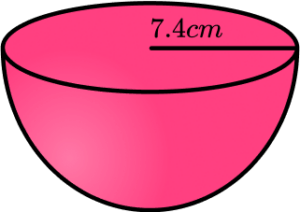588.3 \ cm^2344.1 \ cm^2588.2 \ cm^2392.2 \ cm^2The curved surface area of the hemisphere can be found by using the surface area of a sphere and halving it.

\begin{aligned} \text{Curved surface area}&=4\pi r^2\div 2\\\\ &=4\pi (7.4)^2\div 2\\\\ &=344.067…\\\\ &=344.1 \mathrm{cm}^2 \ \text{(to 1 dp)} \end{aligned}

2. Find the total surface area of a hemisphere with radius 11.3 \ cm. Give your answer correct to 1 decimal place.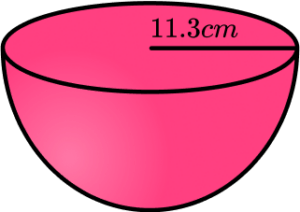401.1 \ cm^2802.3 \ cm^21203.4 \ cm^23209.2 \ cm^2The curved surface area of the hemisphere can be found by using the surface area of a sphere and halving it. We need to add the area of the flat circle as we need the total surface area.

\begin{aligned} \text{Total surface area}&=4\pi r^2\div 2+\pi r^2\\\\ &=3\pi r^2\\\\ &=3\pi (11.3)^2\\\\ &=1203.449…\\\\ &=1203.4 \ \mathrm{cm}^2 \ \text{(to 1 dp)} \end{aligned}

3. Find the total surface area of a hemisphere with diameter 15.3 \ cm. Give your answer correct to 1 decimal place.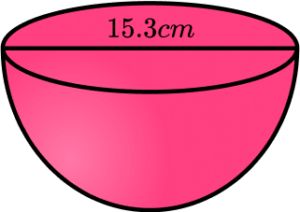588.3 \ cm^21470.8 \ cm^22206.2 \ cm^2551.6 \ cm^2The curved surface area of the hemisphere can be found by using the surface area of a sphere and halving it. We need to add the area of the flat circle as we need the total surface area. But first we need to divide the diameter by 2 to find the radius.

r=15.3\div 2=7.65

\begin{aligned} \text{Total surface area}&=4\pi r^2\div 2+\pi r^2\\\\ &=3\pi r^2\\\\ &=3\pi (7.65)^2\\\\ &=551.561…\\\\ &=551.6 \ \mathrm{cm}^2 \ \text{(to 1 dp)} \end{aligned}

4. Find the curved surface area of a hemisphere with radius 5 \ cm. Leave your answer in terms of \pi.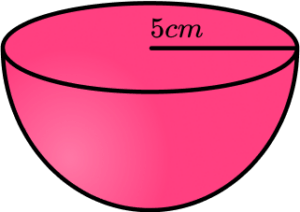25\pi \ cm^250\pi \ cm^275\pi \ cm^2100\pi \ cm^2The curved surface area of the hemisphere can be found by using the surface area of a sphere and halving it.

\begin{aligned} \text{Curved surface area}&=4\pi r^2\div 2\\\\ &=4\pi (5)^2\div 2\\\\ &=50\pi\\\\ &=50\pi \ \mathrm{cm}^2 \end{aligned}

5. Find the total surface area of a hemisphere with radius 11 \ cm. Leave your answer in terms of \pi.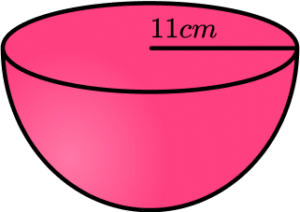363\pi \ cm^2121\pi \ cm^2242\pi \ cm^2484\pi \ cm^2The curved surface area of the hemisphere can be found by using the surface area of a sphere and halving it. We need to add the area of the flat circle as we need the total surface area.

\begin{aligned} \text{Total surface area}&=4\pi r^2\div 2+\pi r^2\\\\ &=3\pi r^2\\\\ &=3\pi (11)^2\\\\ &=363\pi\\\\ &=363\pi \ cm^2 \end{aligned}

6. The total surface area of a hemisphere is 7500 \ cm^{2}. Find the radius of the hemisphere. Give your answer to 3 significant figures.

48.9 \ cm28.2 \ cm69.1 \ cm24.4 \ cmThe total surface area of the hemisphere can be found by using the surface area of a sphere and halving it then adding the area of the flat circle.

This gives 3\pi r^{2}. We can form an equation using the given value and rearrange to solve it.

\begin{aligned} \text{Total surface area}&=4\pi r^2\div 2+\pi r^2\\\\ \text{Total surface area}&=3\pi r^2\\\\ 7500&=3\pi r^2\\\\ 2500&=\pi r^2\\\\ \frac{2500}{\pi}&=r^2\\\\ r&=\sqrt{\frac{2500}{\pi}}\\\\ r&=28.2094…\\\\ r&=28.2 \ cm \ \text{(to 3 sf)} \end{aligned}

### Surface area of a hemisphere GCSE questions

1. A hemisphere has a radius of 13 \ cm.

Calculate the total surface area of the hemisphere.

(3 marks)

CSA: 338\pi, circular base: 169\pi OR 3\pi r^{2}

(1)

Evidence of adding CSA and circular base.

(1)

507\pi \ cm^2

(1)

2. An artist is making a sculpture of a hemisphere with diameter 8 metres.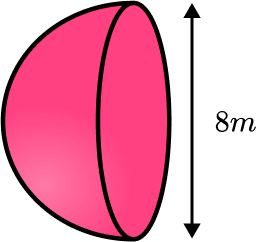He needs to coat the total surface area in paint.

A tin of paint covers an area of 20m^{2}.

Calculate how many tins of paint the artist will need to buy.

(4 marks)

(1)

4\pi (4)^2\div 2+\pi (4)^2 = 3\pi (4)^2 = 48\pi = 150.796…

(1)

48\pi \div 20

(1)

7.539… = 8 tins of paint

(1)

3. A hemisphere has a total surface area of 800 \ cm^{2}.

Calculate its diameter.

(4 marks)

800=3\pi{r}^{2}

(1)

r=\sqrt{\frac{800}{3\pi}}

(1)

9.21317…

(1)

18.4263… = 18.4 \ cm

(1)

## Learning checklist

You have now learned how to:

• Find the curved surface area of a hemisphere
• Find the total surface area of a hemisphere
• Solve problems involving the surface area of a hemisphere

## Still stuck?

Prepare your KS4 students for maths GCSEs success with Third Space Learning. Weekly online one to one GCSE maths revision lessons delivered by expert maths tutors.

Find out more about our GCSE maths tuition programme.